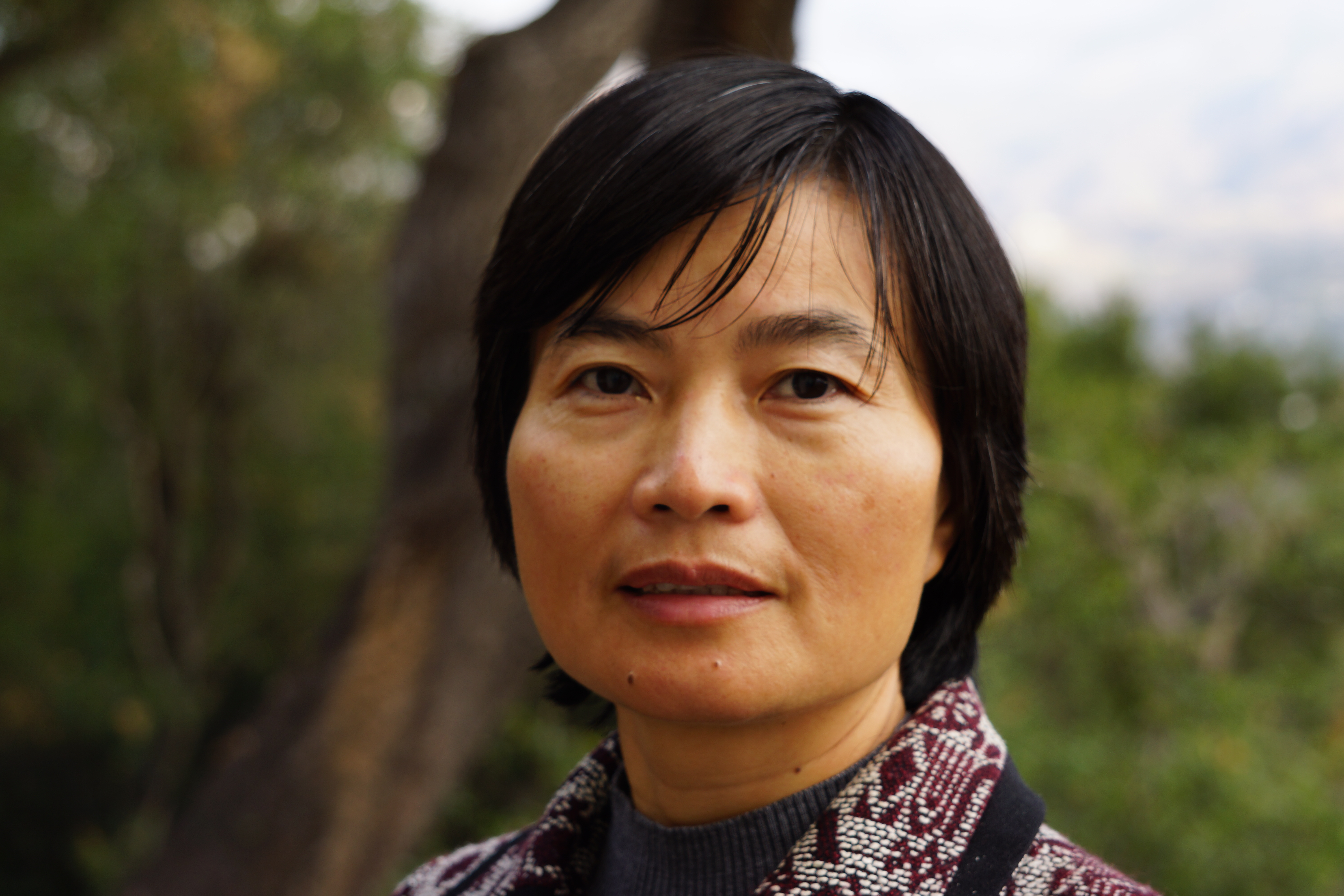## Speaker Biography

Guofang Wei obtained B.S. from Zhejiang University in 1985, Ph.D. from SUNY Stony Brook 1989. She was a Moore Instructor at MIT from 1989-1991 and has been at UC Santa Barbara since 1992. Prof. Wei is a Fellow of the American Mathematical Society, Eisenbud Professor at MSRI, Spring 2016.

## Talk Abstract

Sharp Fundamental Gap Estimate on Convex  Domains of  Shere

In their celebrated work, B. Andrews and J. Clutterbuck proved the fundamental gap (the difference between the first two eigenvalues) conjecture for convex domains in the Euclidean space and conjectured similar results holds for spaces with constant sectional curvature. We prove the conjecture for the sphere. Namely when $D$, the diameter of a convex domain in the unit $S^n$ sphere, is $\le \frac{\pi}{2}$, the gap is greater than the gap of the corresponding $1$-dim sphere model. We also prove the gap is $\ge 3\frac{\pi^2}{D^2}$ when $n \ge 3$, giving a sharp bound. As in B. Andrews and J. Clutterbuck’s work, the key is to prove a super log-concavity of the first eigenfunction. This is a joint work with S. Seto and L. Wang.